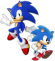To build up your knowledge of C and to eventually solve the here is a set of mini challenges. Write a program in C that reads two numbers, a minutes number and a seconds number, and displays the total seconds. For example, if 14 is read and then 23 is read, the program should display the number 863, because 60*14+23=863. A solution for this challenge is at http://primepuzzle.com/jslinux/chapter.0.c Chapter 1.Sonic the hedgehog takes a total of 863 seconds to run a mile. His pace is 14:23, because 863/60 = 14 minutes, with a remainder of 23 seconds. Write a program in C which reads a number representing total seconds and outputs two numbers, the minutes and the seconds corresponding to the input. Require that the input be less than 1500. A solution for this challenge is at http://primepuzzle.com/jslinux/chapter.1.c Chapter 2. Write a program in C that reads values like the following: 3 14,23 13,19 12,47 The first number is the number of pairs of numbers that follow. The pairs stand for Sonic the hedgehog's running pace in minutes,seconds per mile. Your program should output the fastest pace (in our example it would be 12,47), the slowest pace (14,23) and the average pace. To get the average pace, turn the pairs into seconds, add these seconds, then divide by 3. Drop any partial seconds. Convert this quotient into minutes,seconds. 14*60+23 + 13*60+19 + 12*60+47 = 2429 seconds 2429/3 = 809 seconds (partial seconds dropped) 809 seconds = 13 minutes and 29 seconds A solution for this challenge is at http://primepuzzle.com/jslinux/chapter.2.c Chapter 3.Write a program in C that reads data like 5 3.1,13:25 3.2,13:29 3.3,14:20 3.4,14:10 3.5,10:29 The first number read is the number of pairs of mile mark,pace in mm:ss (minutes and seconds) which follow. Output the average pace, the fastest pace and the mile mark at which it occurred, and the slowest pace and the mile mark at which it occurred. In our example the output would look like Average pace : 13:10 Fastest pace : 10:29 which occurred at mile mark 3.5 Slowest pace : 14:20 which occurred at mile mark 3.3 Note: The exercises in Chapters 4 and 5 will show you how to parse strings and use library functions. Solution: Chapter 4.Write a program in C that reads a line feed delimited text file and outputs each line on a new line. Use the file at http://primepuzzle.com/jslinux/chapter.4.txt to test the program. We have completed this one for you as file reading, pointer usage, and several string functions may be unfamiliar. We will use some of the ideas in this program to solve the exercise in Chapter 3. We will need to parse a long string like ( stands for a newline character) 3.4,14:103.5,13:12 etc. into individual strings 3.4,14:10 and 3.5,13:12 A solution for this challenge is at http://primepuzzle.com/jslinux/chapter.4.c Chapter 5.Libraries contain books, movies etc. Computer programs use libraries too. They are collections of functions. Write a program in C that uses a library defined in a file named library.c that contains a function that displays mm:ss when given total seconds. Example run: D:\jslinux>chapter.5.exe enter seconds 834 mm:ss 13:54 D:\jslinux> Solution: Chapter 6.Arrays may be used to hold a series of related data. Write a program in C to read in a file of line feed delimited data and store the "records" in a character array. Use the file at http://primepuzzle.com/jslinux/chapter.4.txt A simple example of the use of a character array is at http://primepuzzle.com/jslinux/arrays.c Then read in two numbers, n and m, a starting record number and an ending record number. Display these records and the average pace in mm:ss of the records between the nth and mth records (inclusive). Use 0-based indexing. That is, the first record is record 0. Use the ideas in previous chapters to solve the problem. For example: If the n and m are 7 and 12 your output should be 0.7,18:34 0.8,16:40 0.9,16:23 1.0,19:25 1.1,18:39 1.2,19:56 18:16 Solution: Chapter 7.How do you handle command tails in C? This program solves the March Programming Challenge. The file to be read is supplied via a command tail. chapter.7.exe -f filename [-n num] The -n num option tells the program how many consecutive records to average. We print the average pace for each num contiguous records. We print the fastest average pace of any num contiguous records. We print the slowest average pace of any num contiguous records.